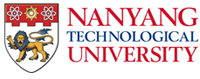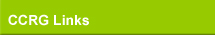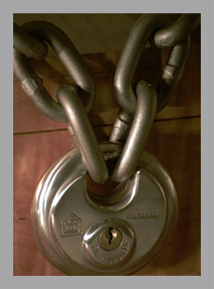The following are sampling of research areas that are of active interest to the Coding & Cryptography Research Group (CCRG). Mathematical Foundations of Coding & Cryptography Applications of algebraic geometry in coding & cryptography Combinatorial designs & blocks codes Quantum error-correcting codes Space-time codes Authentication & digital signature Public-key and symmetric-key cryptography Complexity issues in coding & cryptography Engineering Study of Coding & Cryptography Decoding of algebraic geometry codes & polynomial codes New applications of coding theory Design & analysis of cryptographic algorithms (including block ciphers, stream ciphers & hash functions) Software Implementation of Cryptography Computer, database, network & multimedia security